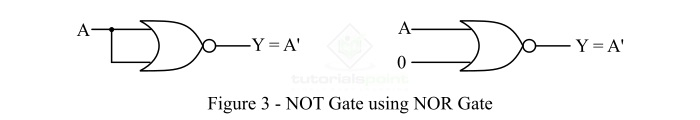# Implementation of NOT Gate from NOR Gate

A NOT Gate is a basic logic gate that gives an output which is complement of its input. A NOR gate is a universal logic gate that can be used to implement any other type of logic gate. A NOR gate is basically an OR gate followed by a NOT gate.

Read this tutorial to find out how you can implement a NOT gate using NOR gate. Let's start the discussion with a brief overview of NOT and NOR gates.

## What is a NOT Gate?

In digital electronics, a NOT gate is a basic logic gate that has only one input and one output. It is type of logic gate whose output is always the complement of its input. Therefore, the NOT gate is also known as an inverter.

If the input of the NOT gate is LOW (Logic 0), then it gives an output that is HIGH (Logic 1). If the input is HIGH (Logic 1), then the NOT gate gives the LOW (logic 0) output. The logic symbol of the NOT gate is shown in Figure-1.The NOT operation is represented by the '-' (bar) symbol. Therefore, if the input variable of the NOT gate is A, then its output Y is given by,

$$\mathrm{Y = \bar{A} = A' }$$

### Truth Table of NOT Gate

The truth table of the NOT gate gives the relationship between its input variable and output variable. The following is truth table of the NOT gate-

Input (A)

Output (Y = A')

A

B

0

1

1

0

## What is a NOR Gate?

NOR gate is a type of universal gate, therefore, it can be used to implement any other type of logic gate. NOR gate is basically a combination of NOT gate and OR gate, i.e. OR gate followed by a NOT gate is a NOR gate. Thus,

$$\mathrm{NOR\:Gate = OR\:Gate = NOT\:Gate }$$

A NOR gate can accept any number of inputs and gives a single output. The output of the NOR gate is assumed HIGH or Logic 1, only when all of its inputs are LOW or Logic 0. For any other combination of inputs, the output of the NOR gate is assumed LOW or Logic 0. The logic symbol of a two input NOR gate is shown in Figure-2.The operation of the NOR Gate is expressed by,

$$\mathrm{Y = \overline{A+B} = (A+B)'}$$

Where, A and B are the input variables and Y is the output variable of the NOR gate. The output expression of the NOR gate is read as "Y is equal to A plus B whole bar".

For different combinations of inputs, we can analyze the operation of the NOR gate using its truth table, which is given below.

Input

Output

A

B

Y = (A + B)'

0

0

1

0

1

0

1

0

0

1

1

0

Now, let us discuss the implementation of NOT Gate using NOR Gate.

## Implementation of NOT Gate from NOR Gate

As discussed above, a NOR gate is a universal gate, therefore, we can use it to realize any other type of logic gate. The implementation of NOT gate from NOR gate is shown in Figure-3.Hence, to realize the NOT gate using NOR gate, we simply join all its inputs terminals together and apply the signal to be NOTed or inverted to this common input terminal.

Also, we can use the NOR gate as a NOT gate by connecting all its input terminals except one to Logic 0, and applying the signal to be inverted to the remaining terminal. This configuration of NOR Gate as NOT Gate is referred to as a controlled inverter.

Hence, in this way, the NOT gate can be implemented using a NOR gate only.

Updated on: 10-Jan-2023

6K+ Views